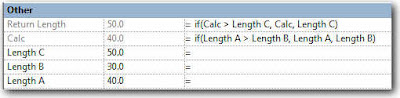Saturday, November 7, 2009

Formula to return the highest value

For the family builders, here´s a quick trick to return the highest of three values (user inputs)

We need something like the following parameters:

Length A (User input)
Length B (User input)
Length C (User input)
Calc (Only used to get our final result)
Return Length (Returns the greatest of the three length parameters)

The formulas needed goes like this:

Calc = if(Length A > Length B, Length A, Length B)

Return Length = if(Calc > Length C, Calc, Length C)Download the sample family from HEREEdit:

While talking about this topic at the Augi Forums, Joe Zhou came up with this genius formula (The result is the same, but you won´t need the "Calc" parameter...

Return Length = if(and(or(Length A > Length B, Length A = Length B), or(Length A > Length C, Length A = Length C)), Length A, if(and(or(Length B > Length A, Length B = Length A), or(Length B > Length C, Length B = Length C)), Length B, if(and(or(Length C > Length A, Length C = Length A), or(Length C > Length B, Length C = Length B)), Length C, 0 mm)))

OK... the formula itself is a nightmare, but it WORKS !